## 7.1 Sampling bowl activity

### 7.1.1 What proportion of this bowl’s balls are red?

Take a look at the bowl in Figure 7.1. It has a certain number of red and a certain number of white balls all of equal size. Furthermore, it appears the bowl has been mixed beforehand, as there does not seem to be any coherent pattern to the spatial distribution of the red and white balls.

Let’s now ask ourselves, what proportion of this bowl’s balls are red?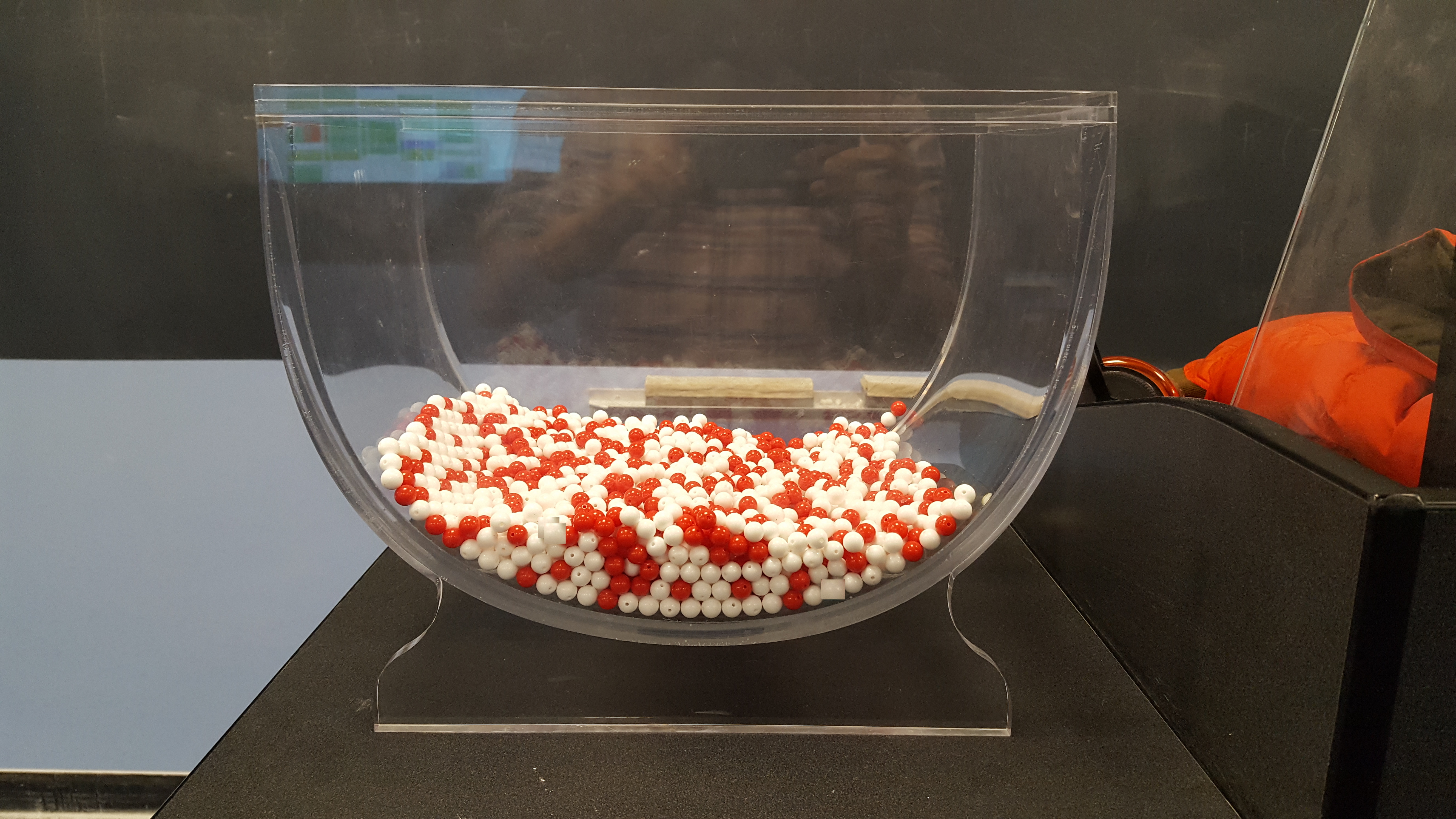FIGURE 7.1: A bowl with red and white balls.

One way to answer this question would be to perform an exhaustive count: remove each ball individually, count the number of red balls and the number of white balls, and divide the number of red balls by the total number of balls. However, this would be a long and tedious process.

### 7.1.2 Using the shovel once

Instead of performing an exhaustive count, let’s insert a shovel into the bowl as seen in Figure 7.2. Using the shovel, let’s remove $$5 \cdot 10 = 50$$ balls, as seen in Figure 7.3.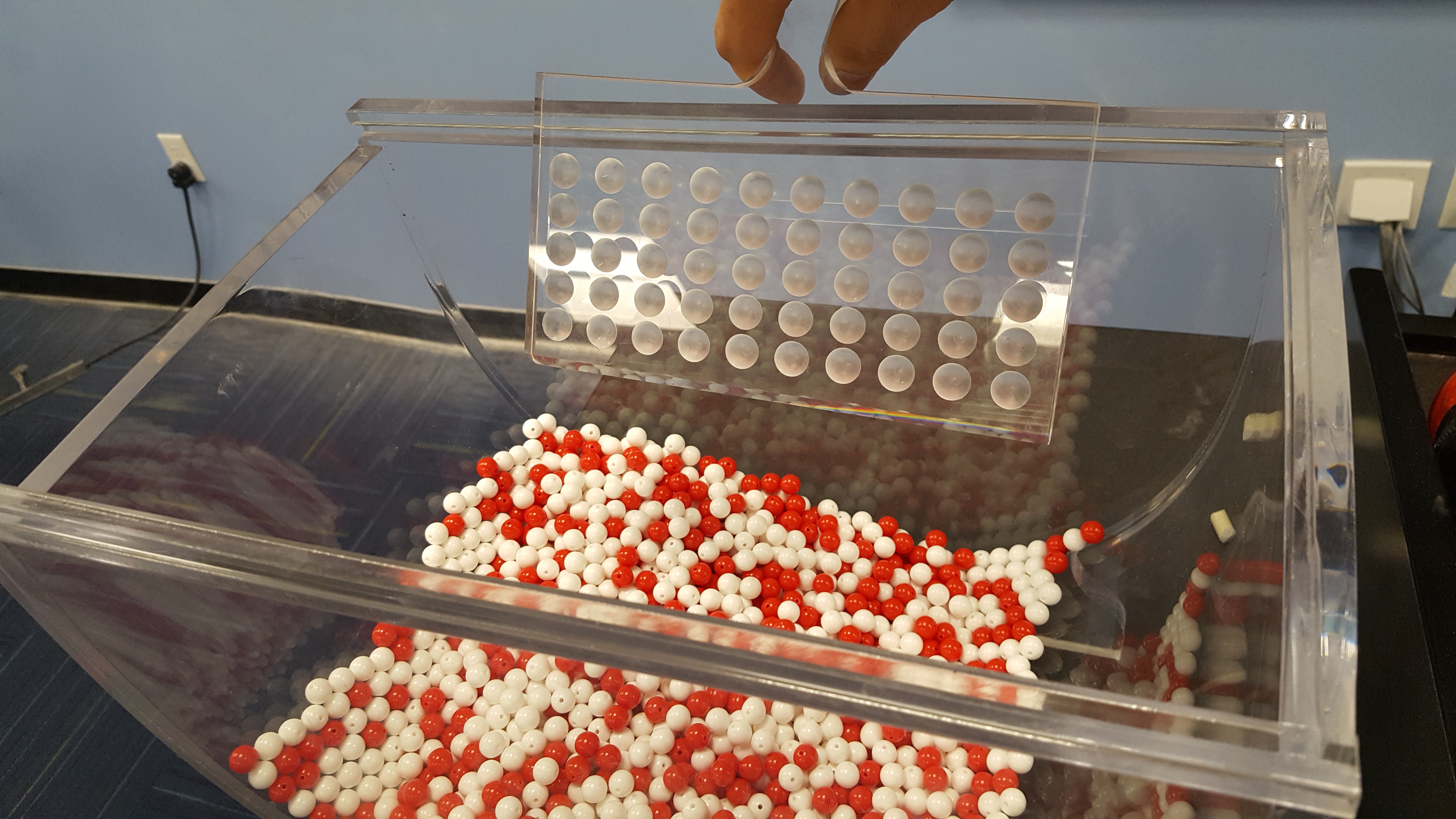FIGURE 7.2: Inserting a shovel into the bowl.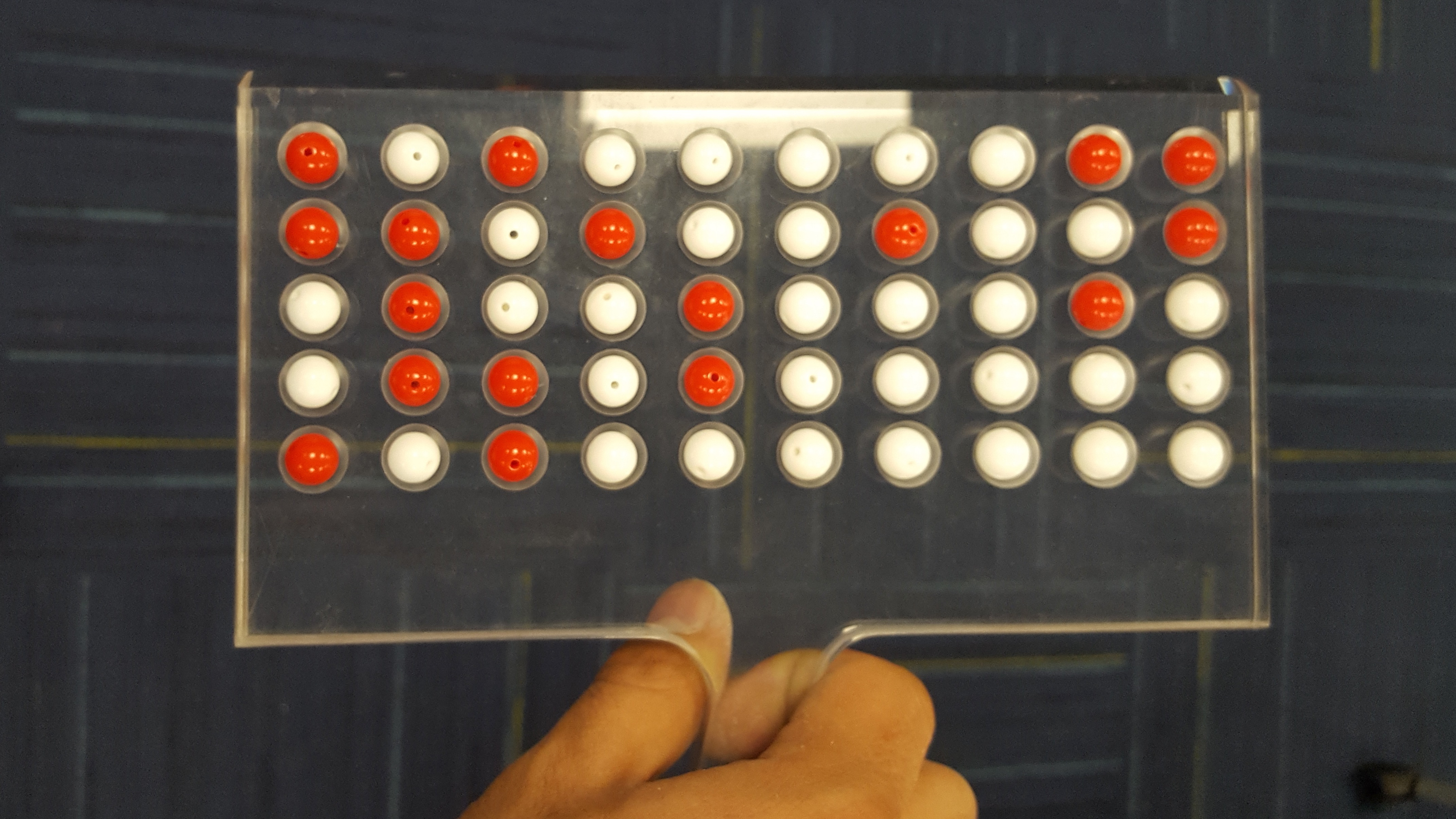FIGURE 7.3: Removing 50 balls from the bowl.

Observe that 17 of the balls are red and thus 0.34 = 34% of the shovel’s balls are red. We can view the proportion of balls that are red in this shovel as a guess of the proportion of balls that are red in the entire bowl. While not as exact as doing an exhaustive count of all the balls in the bowl, our guess of 34% took much less time and energy to make.

However, say, we started this activity over from the beginning. In other words, we replace the 50 balls back into the bowl and start over. Would we remove exactly 17 red balls again? In other words, would our guess at the proportion of the bowl’s balls that are red be exactly 34% again? Maybe?

What if we repeated this activity several times following the process shown in Figure 7.4? Would we obtain exactly 17 red balls each time? In other words, would our guess at the proportion of the bowl’s balls that are red be exactly 34% every time? Surely not. Let’s repeat this exercise several times with the help of 33 groups of friends to understand how the value differs with repetition.

### 7.1.3 Using the shovel 33 times

Each of our 33 groups of friends will do the following:

• Use the shovel to remove 50 balls each.
• Count the number of red balls and thus compute the proportion of the 50 balls that are red.
• Return the balls into the bowl.
• Mix the contents of the bowl a little to not let a previous group’s results influence the next group’s.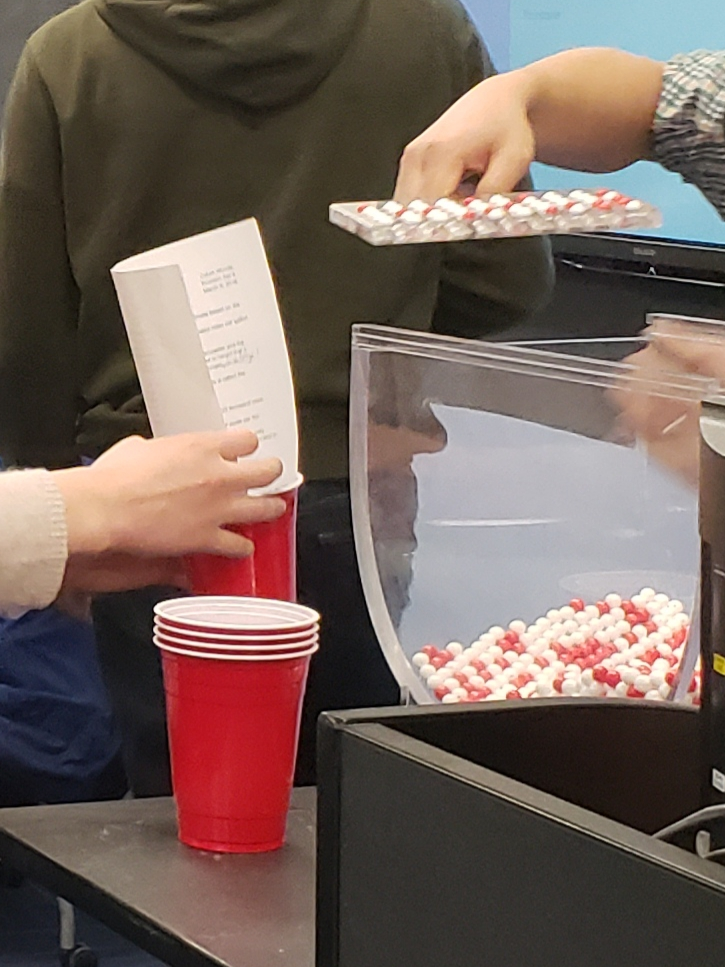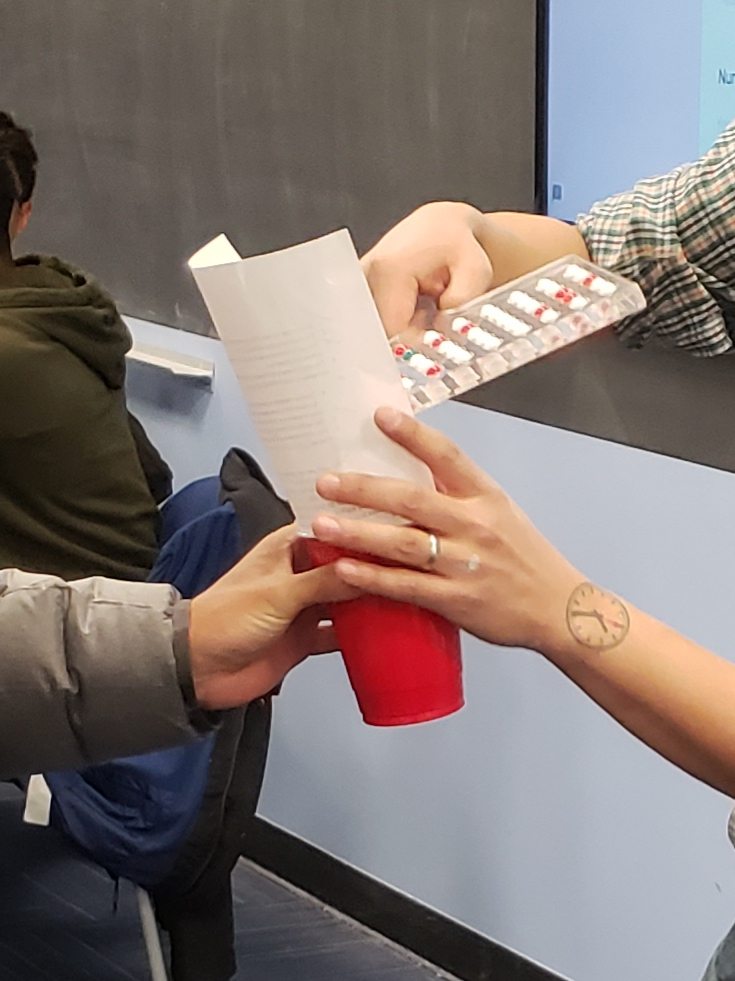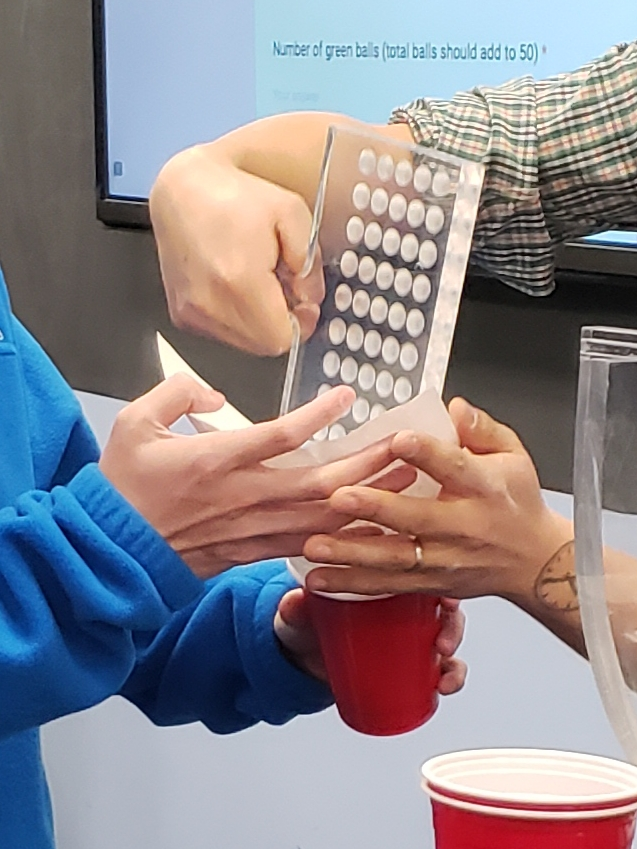FIGURE 7.4: Repeating sampling activity 33 times.

Each of our 33 groups of friends make note of their proportion of red balls from their sample collected. Each group then marks their proportion of their 50 balls that were red in the appropriate bin in a hand-drawn histogram as seen in Figure 7.5.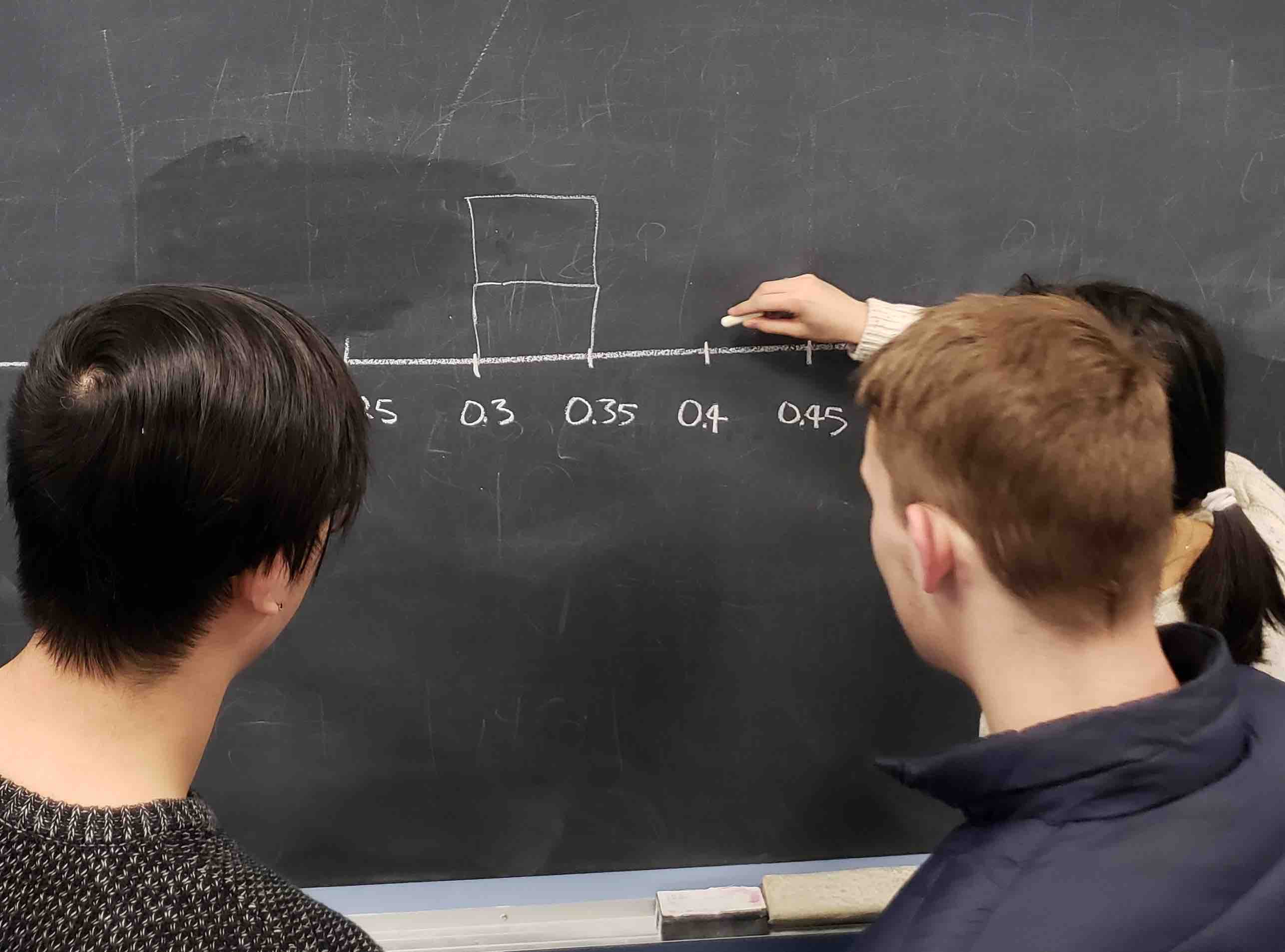FIGURE 7.5: Constructing a histogram of proportions.

Recall from Section 2.5 that histograms allow us to visualize the distribution of a numerical variable. In particular, where the center of the values falls and how the values vary. A partially completed histogram of the first 10 out of 33 groups of friends’ results can be seen in Figure 7.6.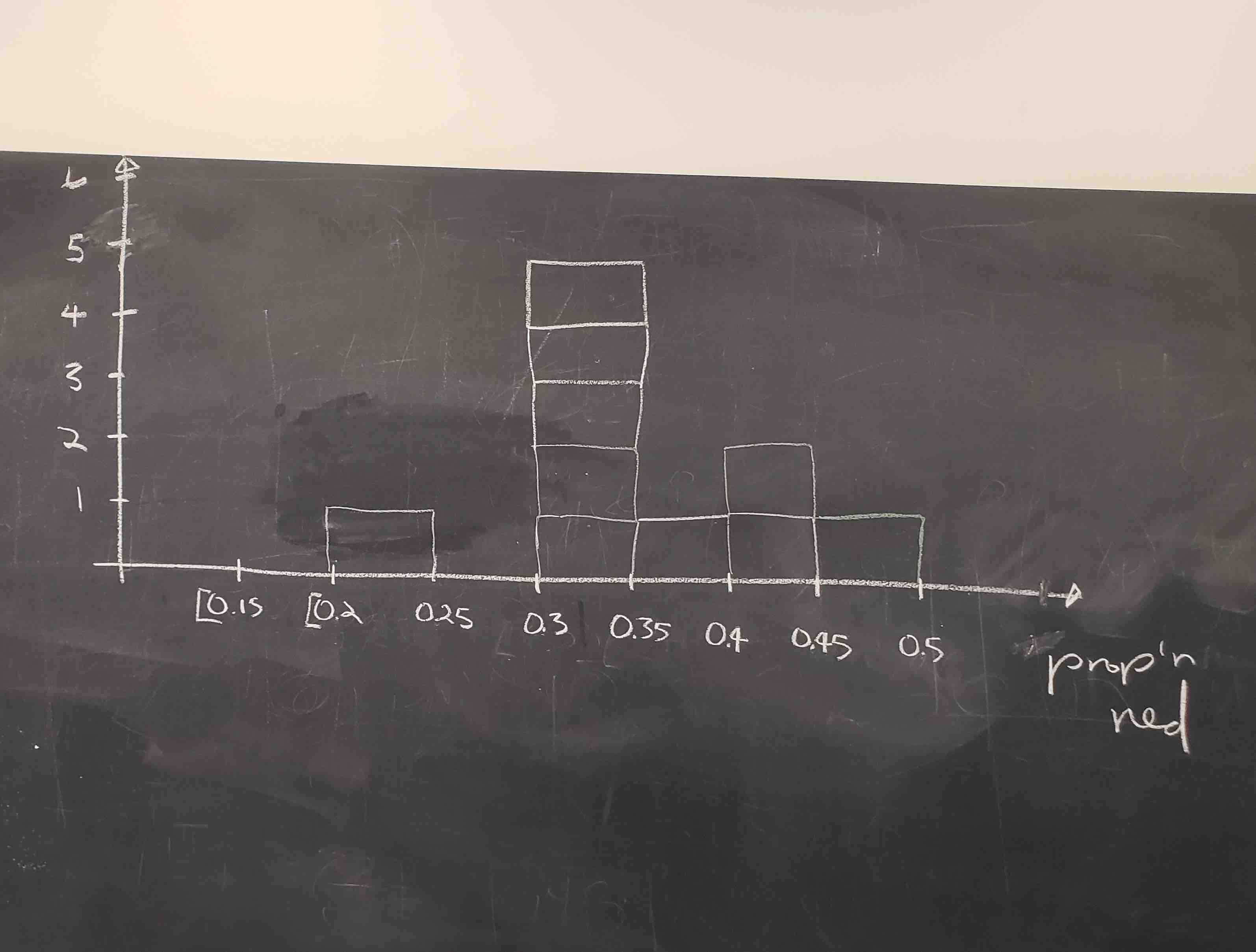FIGURE 7.6: Hand-drawn histogram of first 10 out of 33 proportions.

Observe the following in the histogram in Figure 7.6:

• At the low end, one group removed 50 balls from the bowl with proportion red between 0.20 and 0.25.
• At the high end, another group removed 50 balls from the bowl with proportion between 0.45 and 0.5 red.
• However, the most frequently occurring proportions were between 0.30 and 0.35 red, right in the middle of the distribution.
• The shape of this distribution is somewhat bell-shaped.

Let’s construct this same hand-drawn histogram in R using your data visualization skills that you honed in Chapter 2. We saved our 33 groups of friends’ results in the tactile_prop_red data frame included in the moderndive package. Run the following to display the first 10 of 33 rows:

tactile_prop_red
# A tibble: 33 x 4
group            replicate red_balls prop_red
<chr>                <int>     <int>    <dbl>
1 Ilyas, Yohan             1        21     0.42
2 Morgan, Terrance         2        17     0.34
3 Martin, Thomas           3        21     0.42
4 Clark, Frank             4        21     0.42
5 Riddhi, Karina           5        18     0.36
6 Andrew, Tyler            6        19     0.38
7 Julia                    7        19     0.38
8 Rachel, Lauren           8        11     0.22
9 Daniel, Caroline         9        15     0.3
10 Josh, Maeve             10        17     0.34
# … with 23 more rows

Observe for each group that we have their names, the number of red_balls they obtained, and the corresponding proportion out of 50 balls that were red named prop_red. We also have a replicate variable enumerating each of the 33 groups. We chose this name because each row can be viewed as one instance of a replicated (in other words repeated) activity: using the shovel to remove 50 balls and computing the proportion of those balls that are red.

Let’s visualize the distribution of these 33 proportions using geom_histogram() with binwidth = 0.05 in Figure 7.7. This is a computerized and complete version of the partially completed hand-drawn histogram you saw in Figure 7.6. Note that setting boundary = 0.4 indicates that we want a binning scheme such that one of the bins’ boundary is at 0.4. This helps us to more closely align this histogram with the hand-drawn histogram in Figure 7.6.

ggplot(tactile_prop_red, aes(x = prop_red)) +
geom_histogram(binwidth = 0.05, boundary = 0.4, color = "white") +
labs(x = "Proportion of 50 balls that were red",
title = "Distribution of 33 proportions red")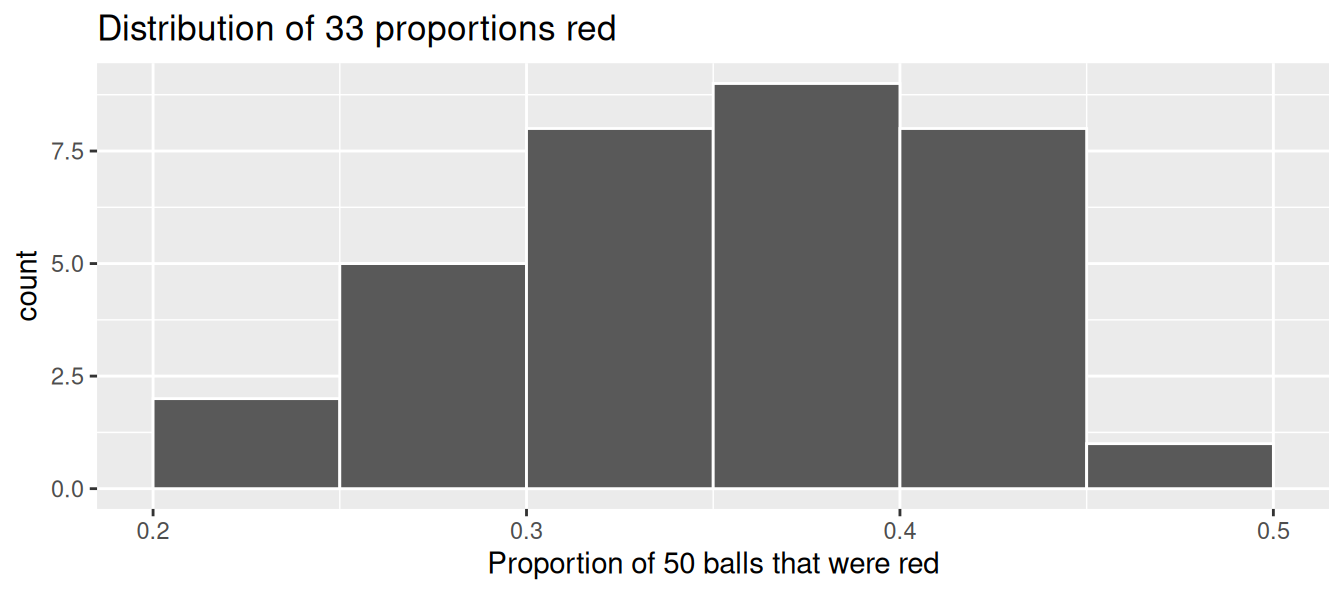FIGURE 7.7: Distribution of 33 proportions based on 33 samples of size 50.

### 7.1.4 What did we just do?

What we just demonstrated in this activity is the statistical concept of sampling. We would like to know the proportion of the bowl’s balls that are red. Because the bowl has a large number of balls, performing an exhaustive count of the red and white balls would be time-consuming. We thus extracted a sample of 50 balls using the shovel to make an estimate. Using this sample of 50 balls, we estimated the proportion of the bowl’s balls that are red to be 34%.

Moreover, because we mixed the balls before each use of the shovel, the samples were randomly drawn. Because each sample was drawn at random, the samples were different from each other. Because the samples were different from each other, we obtained the different proportions red observed in Figure 7.7. This is known as the concept of sampling variation.

The purpose of this sampling activity was to develop an understanding of two key concepts relating to sampling:

1. Understanding the effect of sampling variation.
2. Understanding the effect of sample size on sampling variation.

In Section 7.2, we’ll mimic the hands-on sampling activity we just performed on a computer. This will allow us not only to repeat the sampling exercise much more than 33 times, but it will also allow us to use shovels with different numbers of slots than just 50.

Afterwards, we’ll present you with definitions, terminology, and notation related to sampling in Section 7.3. As in many disciplines, such necessary background knowledge may seem inaccessible and even confusing at first. However, as with many difficult topics, if you truly understand the underlying concepts and practice, practice, practice, you’ll be able to master them.

To tie the contents of this chapter to the real world, we’ll present an example of one of the most recognizable uses of sampling: polls. In Section 7.4 we’ll look at a particular case study: a 2013 poll on then U.S. President Barack Obama’s popularity among young Americans, conducted by Kennedy School’s Institute of Politics at Harvard University. To close this chapter, we’ll generalize the “sampling from a bowl” exercise to other sampling scenarios and present a theoretical result known as the Central Limit Theorem.

Learning check

(LC7.1) Why was it important to mix the bowl before we sampled the balls?

(LC7.2) Why is it that our 33 groups of friends did not all have the same numbers of balls that were red out of 50, and hence different proportions red?